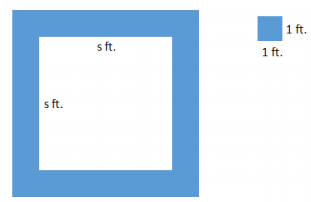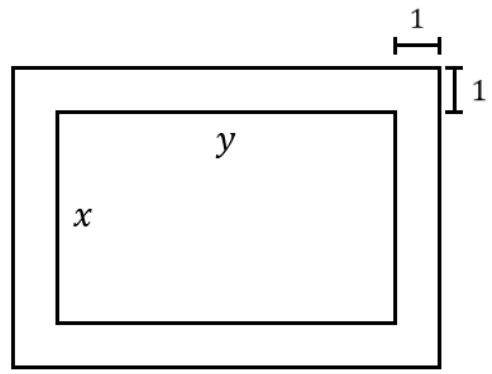Match Fishtank is now Fishtank Learning!

# Numerical and Algebraic Expressions

## Objective

Write and interpret expressions in different ways to shed new meaning on a context.

## Common Core Standards

### Core Standards

?

• 7.EE.A.2 — Understand that rewriting an expression in different forms in a problem context can shed light on the problem and how the quantities in it are related. For example, a + 0.05a = 1.05a means that "increase by 5%" is the same as "multiply by 1.05."

?

• 6.EE.A.3

## Criteria for Success

?

1. Write multiple equivalent expressions to represent a situation.
2. Interpret each expression as it relates to the situation.
3. Understand how different expressions are interpreted differently in a situation.

## Tips for Teachers

?

This lesson touches upon standard 7.EE.2; this standard will be revisited in Unit 5 with percentages and other related contexts.

#### Remote Learning Guidance

If you need to adapt or shorten this lesson for remote learning, we suggest prioritizing Anchor Problem 1 (benefits from discussion). Find more guidance on adapting our math curriculum for remote learning here.

#### Fishtank Plus

• Problem Set
• Student Handout Editor
• Vocabulary Package

## Anchor Problems

?

### Problem 1

Malia is at an amusement park. She bought 14 tickets, and each ride requires 2 tickets.

1. Write an expression that gives the number of tickets Malia has left in terms of $x$, the number of rides she has already gone on. Find at least one other expression that is equivalent to it.
2. The expression ${14}{-2}x$ represents the number of tickets Malia has left after she has gone on $x$ rides. How can each of the following numbers and expressions be interpreted in terms of tickets and rides?
1.   $14$
2.   $-2$
3.   $2x$
3. The expression $2(7-x)$ also represents the number of tickets Malia has left after she has gone on $x$ rides. How can each of the following numbers and expressions be interpreted in terms of tickets and rides?
1.   $7$
2.   $(7-x)$
3.   $2$

#### References

Illustrative Mathematics Ticket to Ride

Ticket to Ride, accessed on Oct. 9, 2017, 11:15 a.m., is licensed by Illustrative Mathematics under either the CC BY 4.0 or CC BY-NC-SA 4.0. For further information, contact Illustrative Mathematics.

### Problem 2

A square fountain area with side length $s$ feet is bordered by a single row of square tiles as shown.What are three different ways to represent the number of tiles needed for the border? Show each representation using the diagram.

#### References

EngageNY Mathematics Grade 7 Mathematics > Module 3 > Topic A > Lesson 3Example 6

Grade 7 Mathematics > Module 3 > Topic A > Lesson 3 of the New York State Common Core Mathematics Curriculum from EngageNY and Great Minds. © 2015 Great Minds. Licensed by EngageNY of the New York State Education Department under the CC BY-NC-SA 3.0 US license. Accessed Dec. 2, 2016, 5:15 p.m..

## Problem Set

?The following resources include problems and activities aligned to the objective of the lesson that can be used to create your own problem set.

• Include any review from previous lessons.

A picture with dimensions $x$ by $y$ inches, is framed by a rectangular border. The border is 1 inch wide, as shown in the figure below.  Write 2 different expressions to represent the area of the border around the picture.